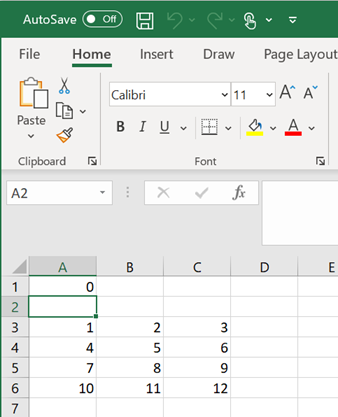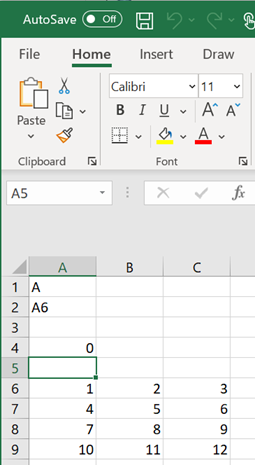MLGetMatrix

Write contents of MATLAB matrix to Microsoft Excel worksheet

Description

example

= MLGetMatrix(var_name,edat) writes the contents of MATLAB® matrix var_name in the Excel® worksheet, beginning in the upper-left cell specified by edat. Use this syntax when working directly in a worksheet.

example

MLGetMatrix var_name, edat writes the contents of MATLAB matrix var_name in the Excel worksheet, beginning in the upper-left cell specified by edat. Use this syntax in a VBA macro.

out = MLGetMatrix(var_name,edat) lets you find errors when you execute the MLGetMatrix function in a VBA macro. If the MLGetMatrix function fails, then out is a string containing an error code. Otherwise, out is 0.

Examples

collapse all

Access the contents of a matrix in MATLAB and write the contents to a worksheet. Specify the name of the matrix and the destination cells explicitly.

Define a 4-by-3 matrix A in MATLAB.

A = [1,2,3;4,5,6;7,8,9;10,11,12]
A =

1     2     3
4     5     6
7     8     9
10    11    12

Open Excel and make sure cell A1 is selected in the worksheet. To write the contents of the MATLAB matrix A starting in cell A3 of worksheet Sheet1, enter this text in cell A1 and press Enter:

= MLGetMatrix("A","Sheet1!A3")

The MLGetMatrix function fills the cells A3 through C6 with data from the matrix.Access the contents of a matrix in MATLAB and write the contents to a worksheet. Specify the destination for the matrix contents by using a cell reference.

Define a 4-by-3 matrix A in MATLAB.

A = [1,2,3;4,5,6;7,8,9;10,11,12]
A =

1     2     3
4     5     6
7     8     9
10    11    12

Open Excel and enter the name of the matrix in cell A1.Enter the reference for the destination cell A6 in cell A2.Make sure cell A4 is selected. Access the MATLAB matrix named by the string in cell A1 and write the contents of the matrix to the worksheet by using the reference in cell A2. Enter this text in cell A4 and press Enter:

= MLGetMatrix(A1,A2)

The MLGetMatrix function fills the cells A6 through C9 with data from the matrix.Access the contents of a matrix in MATLAB and write the contents to a worksheet using a VBA macro.

Define a 4-by-3 matrix A in MATLAB.

A = [1,2,3;4,5,6;7,8,9;10,11,12]
A =

1     2     3
4     5     6
7     8     9
10    11    12

On the Developer tab in Excel, click in the Code group. The Visual Basic® Editor window opens.

From the Insert menu, select Module to insert a new module. In the Module1 window, enter this VBA code containing a macro named GetMatrix.

Sub GetMatrix()
MLGetMatrix "A","B2"
MatlabRequest
End Sub

MatlabRequest initializes internal Spreadsheet Link™ variables and enables MLGetMatrix to work in a subroutine.

The GetMatrix macro uses the MLGetMatrix function to access the contents of matrix A from the MATLAB Workspace. Then, the function writes the contents of the matrix starting at cell B2. For details about working with modules, see Excel Help.

Run the macro by clicking the button on the VBA toolbar. For details about running macros, see Excel Help.

The MLGetMatrix function accesses the contents of the matrix and writes them to the worksheet.

Input Arguments

collapse all

Name of the MATLAB matrix to access, specified as a string. var_name in quotes directly specifies the matrix name. var_name without quotes specifies a worksheet cell address (or range name) that contains the matrix name. Do not use the MATLAB variable ans as var_name.

Example: "A"

Example: A2

Location of the data in the worksheet where the function writes the contents of var_name, specified as a string.

edat in quotes directly specifies the location. edat without quotes specifies a worksheet cell address (or range name) that contains a reference to the location. In both cases, edat must be a cell address or a range name.

Example: "B2"

Example: A6

Tips

• If data exists in the specified worksheet cells, it is overwritten.

• If the dimensions of the MATLAB matrix are larger than that of the specified cells, the data overflows into additional rows and columns.

• edat must not include the cell that contains the MLGetMatrix function. In other words, be careful not to overwrite the function itself. Also make sure the worksheet has enough room to write the matrix contents. If there is insufficient room, the function execution fails because the data has too many rows or columns. For details about the errors in the worksheet, see Worksheet Cell Errors.

• The MLGetMatrix function does not automatically adjust cell addresses. If edat is an explicit cell address, edit it to correct the address when you do either of the following:

• Insert or delete rows or columns.

• Move or copy the function to another cell.

• If worksheet calculation mode is automatic, MLGetMatrix executes when you enter the formula in a cell. If worksheet calculation mode is manual, enter the MLGetMatrix function in a cell, and then press to execute it. However, pressing in this situation may also execute other worksheet functions again and generate unpredictable results.

• If you use MLGetMatrix in a macro subroutine, enter MatlabRequest on the line after the MLGetMatrix command. MatlabRequest initializes internal Spreadsheet Link variables and enables MLGetMatrix to work in a subroutine. Do not include MatlabRequest in a macro function unless the function is called from a subroutine.

• To work with VBA code in Excel with Spreadsheet Link, you must enable Spreadsheet Link as a reference in the Microsoft® Visual Basic Editor. For details, see Installation.

Introduced before R2006a